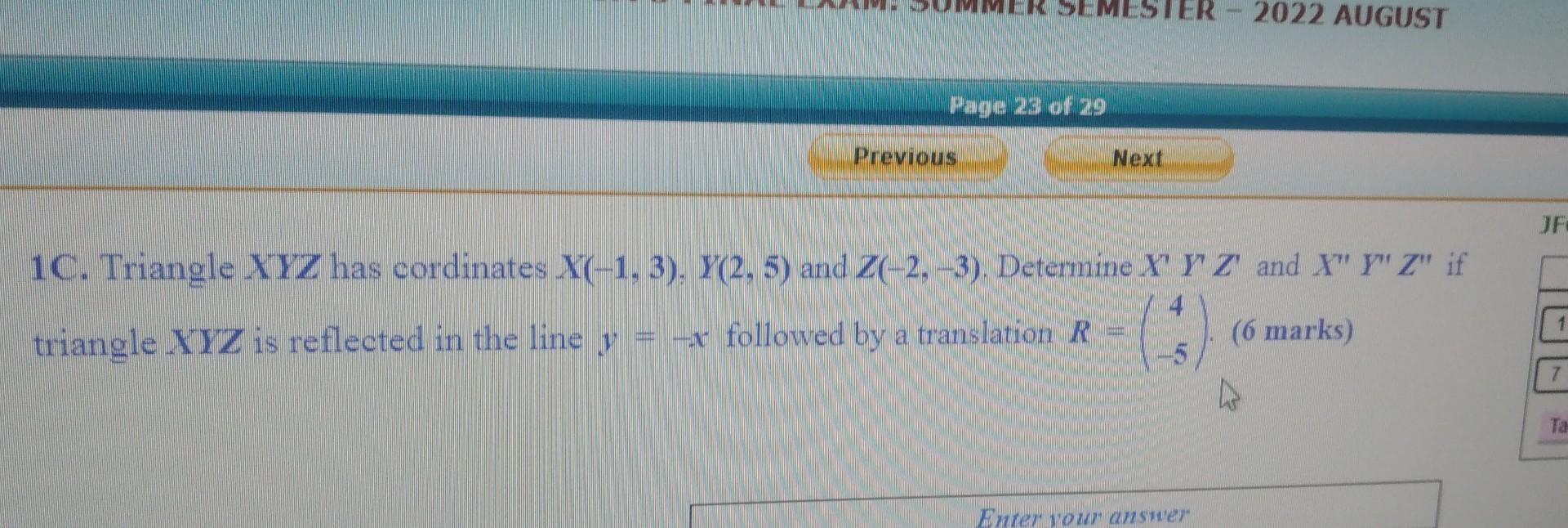# (Solved):   1C. Triangle $$X Y Z$$ has cordinates $$X(-1,3), Y(2,5)$$ and $$Z(-2,-3)$$. Determin ...1C. Triangle $$X Y Z$$ has cordinates $$X(-1,3), Y(2,5)$$ and $$Z(-2,-3)$$. Determine $$X^{\prime} Y^{\prime} Z^{\prime}$$ and $$X^{\prime \prime} Y^{\prime \prime} Z^{\prime \prime}$$ if triangle $$X Y Z$$ is reflected in the line $$y=-x$$ followed by a translation $$R=\left(\begin{array}{c}4 \\ -5\end{array}\right)$$. (6 marks)

We have an Answer from Expert